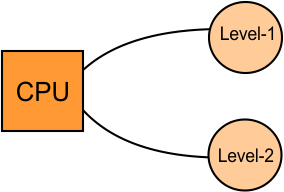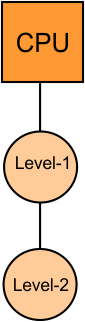Select Page

Computer Organization

Memory Organization
Interrupt
DMA Controller

# Simultaneous Vs. Hierarchical Memory

In the computer, there are two basic methods which are used to access the different levels of memory

1. Simultaneous Access Memory Organization
2. Hierarchical Access Memory Organization

## 1. Simultaneous Access Memory Organization

It is also known as an independent memory organization.

In simultaneous access memory organization, All memory-levels are directly connected with CPU. Whenever CPU requires any word, then it starts searching in all the memory- levels simultaneously to find that particular word.

### Example-01:

When two memory –levels (L1 and L2) are directly connected to the CPU then average access time to any Word and cost per bit is given belowAverage Memory Access Time

Average time required for accessing the memory per operation is given below

H1 x T1 + (1 – H1) x T2

Average Cost Per Byte

The average cost per byte of the memory

= { C1 x S1 + C2 x S2 } / { S1 + S2 }

### Example-02:

When three memory –levels (L1, L2 and L3) are directly connected to the CPU then average access time to any Word and cost per bit is given below

Average Memory Access Time

Average time required to access memory per operation

H1xT1 + (1 – H1) x H2 x T2 + (1 – H1) x (1 – H2) x T3

Average Cost Per Byte

The average cost per byte of the memory

= { C1 x S1 + C2 x S2 + C3 x S3 } / { S1 + S2 + S3 }

## 2. Hierarchical Access Memory Organization

In hierarchical memory organization, all memory levels are connect with each other in a sequence as explain below

• The Level-1 directly connected to the CPU.
• The Level-2 directly connected to level-1.
• and The Level-3 directly connected to level-2 and so on.

Whenever CPU requires any word then,

• It first searches in level1, If the required word is not found in level1 then it look for that word in next level (level2).
• In the same way, If the required word is not found in level 2 then CPU moves to next (level 3) and so on.

### Example-01:

When two memory levels (L1 and L2) are connect to the CPU in hierarchical fashion. Then average access time to any Word and cost per bit, shownAverage Memory Access Time

Average time required to access memory per operation

H1 x T1 + (1 – H1) x (T1 + T2)

Average Cost Per Byte-

Average cost per byte of the memory

= { C1 x S1 + C2 x S2 } / { S1 + S2 }

### Example-02:

When three memory levels (L1, L2 and L3) directly connected to the CPU in hierarchical fashion. Then average access time to any Word and cost per bit, given below

Average Memory Access Time

Average time required to access memory per operation

H1 x T1 + (1 – H1) x H2 x (T1 + T2) + (1 – H1) x (1 – H2) x (T1 + T2 + T3)

Average Cost Per Byte

The average cost per byte of the memory

= { C1 x S1 + C2 x S2 + C3 x S3 } / { S1 + S2 + S3 }

## PRACTICE Questions BASED ON MEMORY ORGANIZATION

### Question – 01:

Consider a system with 2-level caches. Access time of L1, L2 and Main memory are 1ns, 10ns and 500ns. Hit ratio of L1 and L2 are .8 and .9 what is average access time of system ignoring search time within cache?

Solution:

As it is mention in the question ignore the search time in cache. So it is independent memory organization because we cannot ignore the search time in any level in hirerecal memory organization. So

Average Access Time = H1T1 + (1-H1) H2T2 + (1-H1)(1-H2)T3

=0.8×1 + (0.2) x0.9×10 + (0.2)(0.1)x500

=12.6ns

Note: If the memory organization method is not mentioned in the question then first try independent method to solve the problem.

### Problem-02:

Find the average memory access time when cache hit rate of 70% and cache access time of 10 ns and main memory access time of 200 ns through the following cases

1. Independent memory organization.
2. Hierarchical access memory organization

Solution-

Case-01: Independent Memory Organization

Average memory access time

= H1 x T1 + (1 – H1) x T2

= 0.7 x 10 ns + (1 – 0.7)  x 200 ns = 7 ns + 0.3 x 200 ns = 4 ns + 60 ns = 64 ns

Case 02: Hierarchical Access Memory OrganizationAverage memory access time

= H1 x T1 + (1 – H1) x (T1 + T2)

= 0.7 x 10 ns + (1 – 0.7) x (10 ns + 200 ns) = 7 ns + 0.3 x 210 ns = 4 ns + 63 ns = 67 ns

### Problem-03:

Let suppose a computer has a cache, main memory (RAM) and a hard disk used for virtual memory. An access to the cache takes is 1ns, access to main memory takes 10ns and access to the disk takes 1000 ns. Suppose hit ratio of cache is 0.9 and the main memory is 0.8. The effective access time required to access a referenced word on the system is the case when

1. Simultaneous access memory organization is used.
2. Hierarchical access memory organization is used.

Solution-

Part-01:Simultaneous Access Memory Organization-

The memory organization will be as shown-

Effective memory access time

= H1 x T1 + (1 – H1) x H2 x T2 + (1 – H1) x (1 – H2) x H3 x T3

= 0.9 x 1 ns + (1 – 0.9) x 0.8 x 10 ns + (1 – 0.9) x (1 – 0.8) x 1 x 1000 ns = =?

Part-02: Hierarchical Access Memory Organization-

The memory organization will be as shown-

Effective memory access time

= H1 x T1 + (1 – H1) x H2 x (T1 + T2) + (1 – H1) x (1 – H2) x H3 x (T1 + T2 + T3)

= 0.9 x 1 ns + (1 – 0.9) x 0.8 x (1 ns + 10 ns) + (1 – 0.9) x (1 – 0.8) x 1 x (1 ns + 10 ns + 1000 ns) =?

Help Other’s By Sharing…